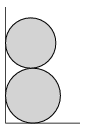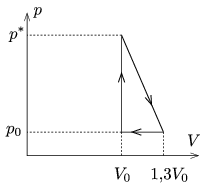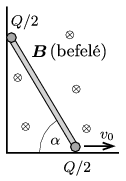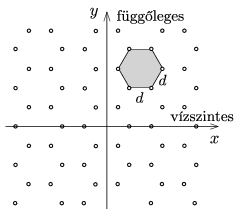Mathematical and Physical Journal
for High Schools
Issued by the MATFUND Foundation
 Already signed up? New to KöMaL?

# KöMaL Problems in Physics, April 2016

Show/hide problems of signs:## Problems with sign 'M'

Deadline expired on May 10, 2016.

M. 359. With measurement determine the amount of carbon-dioxide in a soda charger. (For safety reasons the charger must only be pierced with the pin in the port of the head of the siphon bottle. Take care when you carry out the experiment.)

(6 pont)

solution, statistics## Problems with sign 'P'

Deadline expired on May 10, 2016.

P. 4830. A 2 kg object is moving at a speed of 1.41 m/s in the horizontal plane towards the direction of the north-east. Then for 10 seconds a 0.20 N force is exerted on it towards the west. Determine the magnitude and the direction of the final velocity of the object.

(3 pont)

solution, statistics

P. 4831. A satellite revolves above the equator, at a height of 600 km. What is its period?

(3 pont)

solution, statistics

P. 4832. The frequency of sound wave travelling in air at a speed of 320 m/s is 400 Hz. What is the phase difference between two points which are at a distance of 1 m in the direction of the propagation of sound?

(3 pont)

solution, statistics

P. 4833. During a road repair an old water pipe was found. How can it be decided whether the pipe is used or not without cutting the pipe, and if it is still used how can be the direction of the water flow determined?

(3 pont)

solution, statistics

P. 4834. A uniform density cuboid of edges $\displaystyle a<b<c$ is on the horizontal ground such that its base is its smallest face. The object is turned over about the edge $\displaystyle b$ (which edge remains at rest) such that the least work is performed. This work is 25 times less than the greatest kinetic energy of the cuboid during the turnover. Determine the ratio of the greatest and the smallest edges.

(4 pont)

solution, statistics

P. 4835. Two tables are placed next to each other. The surface of one of them is rough and the other's is very smooth. Two alike springs are attached to the tables and to the other ends of the springs two alike objects are attached. (The springs are designed both for compression and tension.) The object on the rough table is displaced by some distance and released. At the same moment the object on the smooth table is given some initial velocity in the direction of the symmetry axis of the spring. Where will the object on the smooth table be when the other stops moving due to friction? (Air resistance is negligible.)

(5 pont)

solution, statistics

P. 4836. Two uniform rods of equal mass are carefully placed next to a vertical wall horizontally such that one is on the top of the other as shown in the figure. Then the two rods are released. What will the ratio of the greatest speeds of the rods be if the radius of the above rod is just a tiny bit smaller than that of the bottom one? (Friction is negligible everywhere.)(5 pont)

solution, statistics

P. 4837. The cyclic process of a thermodynamic heat engine is shown in the figure. What should the least pressure $\displaystyle p^*$ be in order that the efficiency of he heat engine is maximum, if the working substance is some ideal noble gas of constant mass? What is this maximum efficiency?(5 pont)

solution, statistics

P. 4838. A metal sphere of radius 10 cm is mounted to an insulating stand and is connected (with some conducting material) to an electro-meter of negligible capacitance. The meter reads 15 kV. Another uncharged metal sphere (also mounted to an insulating stand), which is far from the first one, is connected to the charged sphere with thin a piece of wire. Then the reading on the meter is 10 kV. What is the radius of the initially uncharged sphere?

(4 pont)

solution, statistics

P. 4839. A solenoid is made by winding tightly (in one layer) a piece of enamelled wire of resistivity $\displaystyle \varrho$, and of radius $\displaystyle r\ll R$ around an insulating cylinder of length $\displaystyle \ell$ and of radius $\displaystyle R\ll \ell$. The solenoid is connected to a voltage supply of voltage $\displaystyle U$. To what power of the radius $\displaystyle r$ are the following quantities proportional?

$\displaystyle a)$ The current in the wire;

$\displaystyle b)$ the self-inductance of the solenoid;

$\displaystyle c)$ the magnitude of the magnetic induction at the geometric centre of the solenoid.

(4 pont)

solution, statistics

P. 4840. A thin rod of length $\displaystyle L$ is moved in a vertical plane in a right corner such that the speed of the lower, horizontally moving end of the rod has a constant speed of $\displaystyle v_0$, and the other end touches the vertical wall at every moment. At both ends of the rod there are two small charged spheres of charge $\displaystyle Q/2$ (one ball at each end). The whole system is in uniform magnetic field of magnetic induction $\displaystyle \boldsymbol B$. The magnetic induction is horizontal and perpendicular to the plane of the figure pointing into the paper.$\displaystyle a)$ What is the magnitude and the direction of the force exerted on the rod by the magnetic field, when the rod makes an angle of $\displaystyle \alpha$ with the horizontal?

$\displaystyle b)$ Determine this force in the same position of the rod, if there are no charged spheres at the ends of the rod, but there is only one charged sphere at the midpoint of the rod. The charge on this sphere is $\displaystyle Q$.

(5 pont)

solution, statistics

P. 4841. In case of the Compton effect stationary'' electrons are bombarded'' by photons which photons have the same energy as the rest energy of electrons. Among the above described photons there will be some whose linear momentum will be equal in magnitude to the magnitude of the linear momentum of the electrons with which the photons collided. Considering these cases determine the

$\displaystyle a)$ angle between the scattered photons and the electrons with which the photons collided;

$\displaystyle b)$ The speed of the electrons with which the photons collided.

(5 pont)

solution, statistics

P. 4842. There are small holes on an opaque sheet, the holes positioned in a regular hexagonal grid as shown in the figure. The sheet is illuminated by monochromatic laser light of wavelength $\displaystyle \lambda$ perpendicularly to the sheet.What kind of diffraction pattern can be observed on the screen which is placed at a distance of $\displaystyle L$ from the sheet, if the distance between the holes is $\displaystyle d$? What can be stated about the brightness of the peak intensities with respect to each other? (It can be assumed that $\displaystyle L\ll d \ll \lambda$.)

(6 pont)

solution, statistics